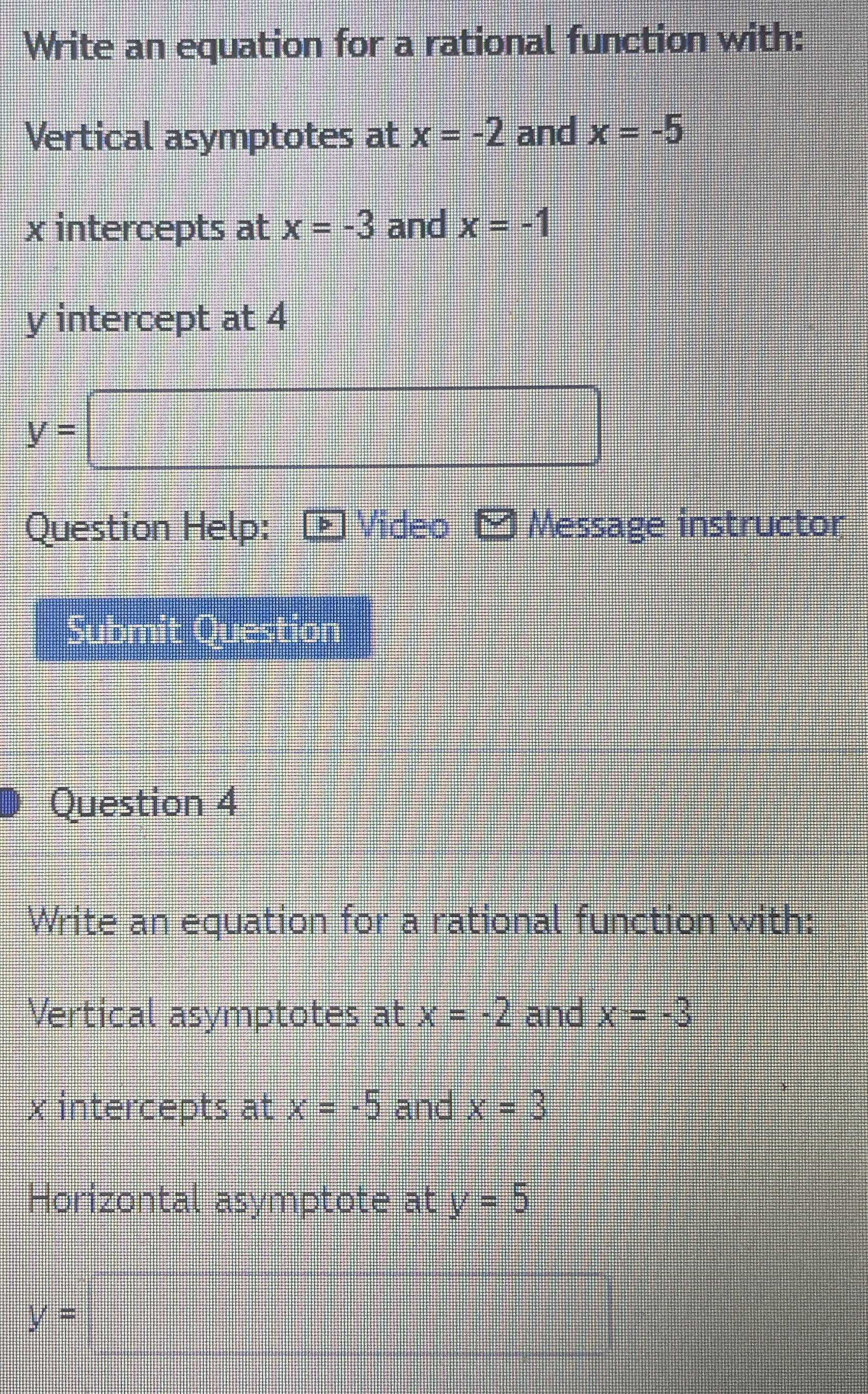### ¿Todavía tienes preguntas de matemáticas?

Pregunte a nuestros tutores expertos
Algebra
Pregunta1. Write an equation for a rational function with: Vertical asymptotes at $$x = - 2$$ and $$x = - 5$$

$$x$$ intercepts at $$x = - 3$$ and $$x = - 1$$

$$y$$ intercept at $$4$$

$$y =$$ $$\square$$

2. Write an equation for a rational function with: Verticat asymptotes at $$x = - 2$$ and $$x = - 3$$

$$x$$ intercepts at $$x = - 5$$ and $$x = 3$$ Horizontal asymptote at $$y = 5$$

$$y =$$ $$\square$$

3. $$f(x)= \frac{6(x+ 2)(x+ 5)}{5(x+ 3)(x+ 1)}$$
4. $$f(x)= \frac{5(x+ 2)(x+ 3)}{(x+ 5)(x- 3)}$$